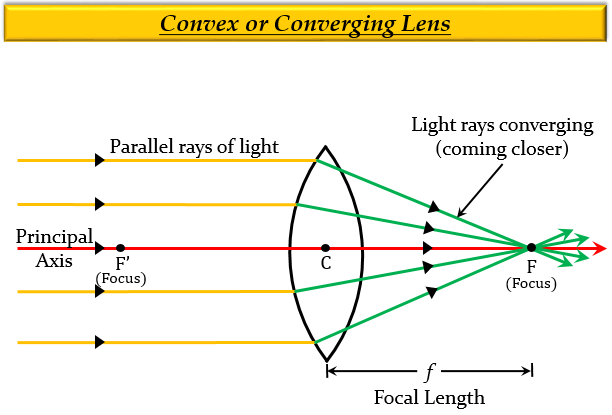# (a) Explain with the help of a diagram, why the convex lens is also called a converging lens.(b) Define principal axis, principal focus and focal length of a convex lens.

(a) A convex lens is also called a converging lens because it converges a parallel beam of light rays passing through it. Think of a magnifying glass that is used to burn something, when the light passing through it concentrates to a point. Look at the diagram given below.(b) Principle axis: It is a line that passes through the optical center of the lens and perpendicular to both the faces of the lens.

Principle focus: It is a point on the principal axis to which light rays parallel to the principal axis converge after passing through the lens.

Focal length: It is the distance between the optical centre and the principal focus of the lens.

Updated on: 10-Oct-2022

396 Views